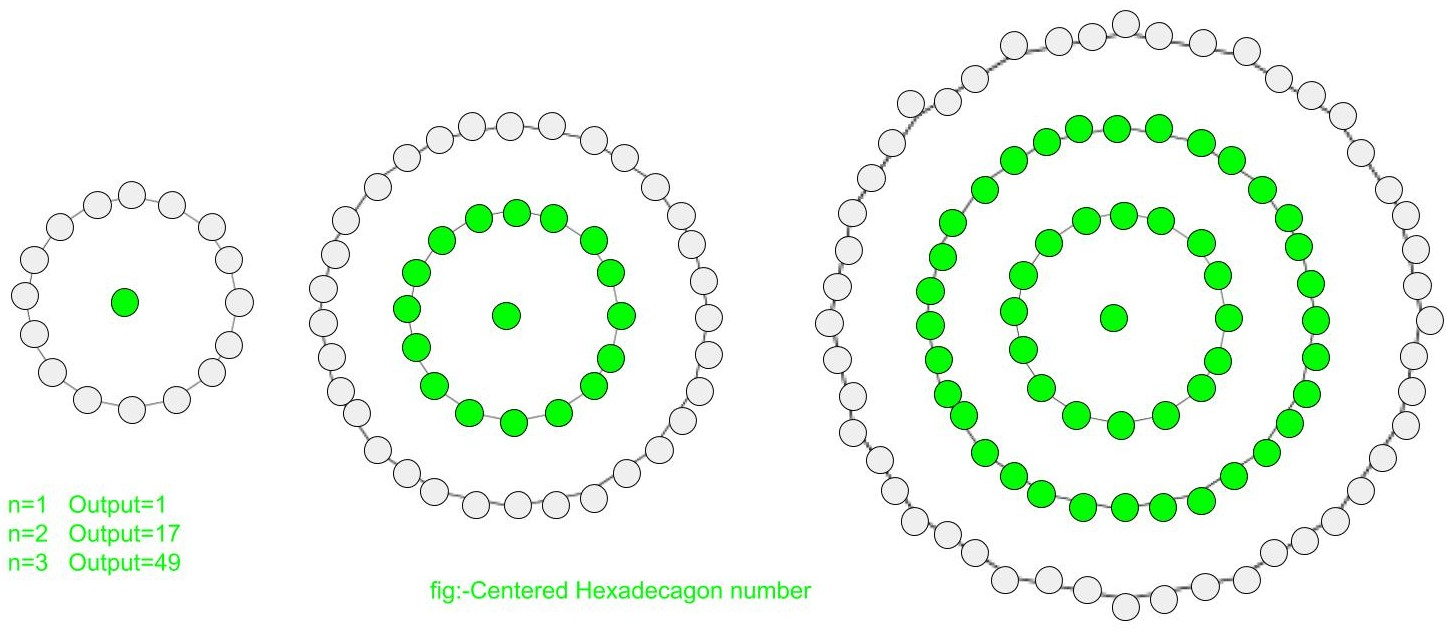Given a number n, find the nth Centered Hexadecagonal Number.

A Centered Hexadecagonal number represents a dot in the centre and other dots around it in successive hexadecagonal(16 sided polygon) layers.The first few Centered Hexadecagonal numbers are:
1, 17, 49, 97, 161, 241, 337, 449, 577, 721, 881………………….

Examples :

```Input :  3
Output : 49

Input : 10
Output : 721
```

## Recommended: Please try your approach on {IDE} first, before moving on to the solution.

In mathematics, Centered hexadecagonal number for the n-th term is given by :

``

Below is the basic implementation of the above idea:

## C++

 `// C++ Program to find ` `// nth centered hexadecagonal ` `// number ` `#include ` `using` `namespace` `std; ` ` `  `// centered hexadecagonal function ` `int` `center_hexadecagonal_num(``long` `int` `n) ` `{ ` `    ``// Formula to calculate nth ` `    ``// centered hexadecagonal number ` `    ``return` `8 * n * n - 8 * n + 1; ` `} ` ` `  `// Driver Code ` `int` `main() ` `{ ` `    ``long` `int` `n = 2; ` `    ``cout << n << ``"th centered hexadecagonal number : "`  `                    ``<< center_hexadecagonal_num(n); ` `    ``cout << endl; ` `    ``n = 12; ` `    ``cout << n << ``"th centered hexadecagonal numbe : "` `                    ``<< center_hexadecagonal_num(n); ` ` `  `    ``return` `0; ` `} `

## Java

 `// Java Program to find nth ` `// centered hexadecagonal number ` `import` `java.io.*; ` ` `  `class` `GFG  ` `{ ` `    ``// centered hexadecagonal function ` `    ``static` `int` `center_hexadecagonal_num(``int` `n) ` `    ``{ ` `        ``// Formula to calculate nth ` `        ``// centered hexadecagonal number ` `        ``return` `8` `* n * n -  ` `               ``8` `* n + ``1``; ` `    ``} ` `     `  `    ``// Driver Code ` `    ``public` `static` `void` `main(String args[]) ` `    ``{ ` `        ``int` `n = ``2``; ` `        ``System.out.print(n + ``"th centered "` `+ ` `                    ``"hexadecagonal number: "``); ` `        ``System.out.println(center_hexadecagonal_num(n)); ` `         `  `        ``n = ``12``; ` `        ``System.out.print(n + ``"th centered "` `+ ` `                    ``"hexadecagonal number: "``); ` `        ``System.out.println(center_hexadecagonal_num(n)); ` `    ``} ` `} ` ` `  `// This code is contributed by ajit. `

## Python3

 `# Program to find nth  ` `# centered hexadecagonal  ` `# number ` ` `  `# centered hexadecagonal  ` `# function ` `def` `center_hexadecagonal_num(n): ` `     `  `    ``# Formula to calculate  ` `    ``# nth centered hexadecagonal ` `    ``# number ` `    ``return` `8` `*` `n ``*` `n ``-` `8` `*` `n ``+` `1` ` `  `# Driver Code ` `if` `__name__ ``=``=` `'__main__'` `: ` `         `  `    ``n ``=` `2` `    ``print``(n,``"nd centered hexadecagonal "` `+`  `                              ``"number : "``, ` `              ``center_hexadecagonal_num(n)) ` `    ``n ``=` `12` `    ``print``(n,``"th centered hexadecagonal "` `+`  `                              ``"number : "``, ` `              ``center_hexadecagonal_num(n)) ` `                 `  `# This code is contributed ` `# by akt_mit `

## C#

 `// C# Program to find nth ` `// centered hexadecagonal number ` `using` `System; ` ` `  `class` `GFG ` `{ ` `     `  `    ``// centered hexadecagonal ` `    ``// function ` `    ``static` `int` `center_hexadecagonal_num(``int` `n) ` `    ``{ ` `        ``// Formula to calculate nth ` `        ``// centered hexadecagonal number ` `        ``return` `8 * n * n -  ` `               ``8 * n + 1; ` `    ``} ` `     `  `    ``// Driver Code ` `    ``static` `public` `void` `Main () ` `    ``{ ` `        ``int` `n = 2; ` `        ``Console.Write(n + ``"th centered "` `+ ` `                    ``"hexadecagonal number: "``); ` `        ``Console.WriteLine(center_hexadecagonal_num(n)); ` `         `  `        ``n = 12; ` `        ``Console.Write(n + ``"th centered "` `+ ` `                    ``"hexadecagonal number: "``); ` `        ``Console.WriteLine(center_hexadecagonal_num(n)); ` `    ``} ` `} ` ` `  `// This code is contributed by m_kit `

## PHP

 ` `

Output :

```2th centered hexadecagonal number : 17
12th centered hexadecagonal numbe : 1057
```

References:
http://oeis.org/A069129

Attention reader! Don’t stop learning now. Get hold of all the important DSA concepts with the DSA Self Paced Course at a student-friendly price and become industry ready.

My Personal Notes arrow_drop_upCheck out this Author's contributed articles.

If you like GeeksforGeeks and would like to contribute, you can also write an article using contribute.geeksforgeeks.org or mail your article to contribute@geeksforgeeks.org. See your article appearing on the GeeksforGeeks main page and help other Geeks.

Please Improve this article if you find anything incorrect by clicking on the "Improve Article" button below.

Improved By : jit_t

Article Tags :
Practice Tags :

Be the First to upvote.

Please write to us at contribute@geeksforgeeks.org to report any issue with the above content.